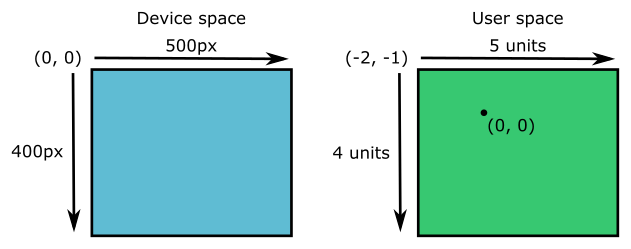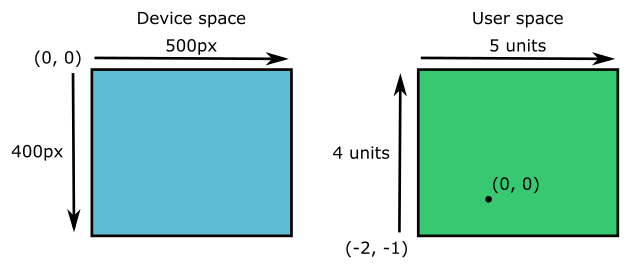# generativepy.drawing module

Martin McBride, 2020-07-27
Tags drawing
Categories generativepy generative artThe generativepy.drawing module contains image creation functions, for creating PNG and SVG images, and frames.

## make_image

Used to create a single PNG image.

```generativepy.drawing.make_image(outfile, draw, width, height, channels=3)
```
Parameter Type Description
outfile String The path and filename for the output PNG file. It should end in '.png'.
draw Function A drawing function object, see below.
width int The width of the image that will be created, in pixels.
height int The height of the image that will be created, in pixels.
channels int The number of colour channels. 3 for RGB, 4 for RGBA

The `channels` parameter controls the transparency of the output file. To create PNGs with a transparent background, you should use RGBA.

Objects can be drawn with transparency even if RGB is used, but the image background will always be opaque unless RGBA is specified.

`make_image` creates a Pycairo surface and context, then calls the user supplied `draw` function to do the drawing.

The draw function must have the following signature:

```draw(ctx, pixel_width, pixel_height, frame_no, frame_count)
```
Parameter Type Description
ctx Context A Pycairo context that the draw function should draw on.
pixel_width int The width of the image in pixels. This is the same value as the width parameter in make_image.
pixel_height int The height of the image in pixels. This is the same value as the height parameter in make_image.
frame_no int The number of the current frame.
frame_count int The total number of frames.

`frame_no` and `frame_count` only apply to functions that create a sequence of images (such as `make_images` below). The `make_image` function only ever creates one image, so the `frame_no` will always be 0 and the `frame_count` will always be 1.

## make_images

Used to create a sequence of PNG images. These can be combined into an animated GIF or video.

```generativepy.drawing.make_images(outfile, draw, width, height, count, channels=3)
```
Parameter Type Description
outfile String The path and base filename for the output PNG files. See below.
draw Function A drawing function object, see `make_image`.
width int The width of each image that will be created, in pixels.
height int The height of each image that will be created, in pixels.
count int The total number of images that will be created.
channels int The number of colour channels. 3 for RGB, 4 for RGBA

The `outfile` parameter should be a base filepath. For example:

```C:/temp/image
```

In this case the files will be created in the folder C:/temp, and their names will be based on the base name "image":

```image00000000.png
image00000001.png
image00000002.png
etc
```

Once the images have been created, you can use an external program to convert them into an animated GIF or movie.

The draw function has the same signature as for `make_image`. In this case though, it will be called `count` times. The `frame_count` will always be set to `count`, and the `frame_no` will increment each time draw is called. You can use the `frame_no` value to draw a different image each time, to create animation.

## make_image_frame

Used to create a single frame.

```generativepy.drawing.make_image_frame(draw, width, height, channels=3)
```
Parameter Type Description
draw Function A drawing function object, see below.
width int The width of the image that will be created, in pixels.
height int The height of the image that will be created, in pixels.
channels int The number of colour channels. 3 for RGB, 4 for RGBA

This works in a similar way to `make_image`, but instead of saving the image to a file, it returns a frame.

## make_image_frames

Used to create a sequence of frames.

```generativepy.drawing.make_image_frames(draw, width, height, count, channels=3)
```
Parameter Type Description
draw Function A drawing function object, see `make_image`.
width int The width of each image that will be created, in pixels.
height int The height of each image that will be created, in pixels.
count int The total number of images that will be created.
channels int The number of colour channels. 3 for RGB, 4 for RGBA

This works in a similar way to `make_images`, but instead of saving the images to a set of files, it returns a lazy sequence of frames.

## make_svg

Used to create a single SVG image. This is similar to `make_image` but it creates a vector SVG image that can be edited in Inkscape and other programs.

```generativepy.drawing.make_svg(outfile, draw, width, height)
```
Parameter Type Description
outfile String The path and filename for the output SVG file. It should end in '.svg'.
draw Function A drawing function object, see below.
width int The width of the image that will be created, in pixels.
height int The height of the image that will be created, in pixels.

There is no `channels` parameter because all SVG files support background transparency.

`make_svg` creates a Pycairo surface and context, then calls the user supplied `draw` function to do the drawing.

The draw function works in the same way as for `make_image`.

## setup

A simple helper function to initialise a page. It is optional, but if you use it it should normally be the first thing you call in your `draw` function.

```setup(ctx, pixel_width, pixel_height, width=None, height=None, startx=0, starty=0,
background=None, flip=False):
```
Parameter Type Description
ctx Context Pycairo context. Use the value passed into `draw`.
pixel_width int The width of the image in pixels. Use the value passed into `draw`.
pixel_height int The height of the image in pixels. Use the value passed into `draw`.
width float The required image width in user coordinates.
height float The required image height in user coordinates.
startx float The user space x coordinate of the device space origin.
starty float The user space y coordinate of the device space origin.
background Color The colour of the background fill.
flip bool If true, flips the page in the y direction, so the origin is at the bottom left, useful for mathematical drawing.

This function maps performs a scaling to set the drawing coordinates. This is optional, but in generative art you will often be using functions that work at a particular scale. It is very useful to be able to set your drawing coordinates to maths this, so you don't need to worry about scaling values when you draw.

As a convenience it can also set the page background colour.

## Device and user space

Pycairo uses the concept of device space and user space to perform scaling:

• Device space represents the actual pixels in the final image.
• User space is the coordinate system you use to draw in.

By default, device space and user space are identical. So if you create a 500 by 400 pixel image, your user space will also be 500 by 400 units. The origin for both spaces is in the top left corner, so y coordinates increase as you move down from the top. This is very common in computer graphics, although it is the opposite of how graphs work in mathematics.

You can change user space using `setup`. For example:

```setup(ctx, pixel_width=500, pixel_height=400, width=5, startx=-2.0, starty=-1.0,
background=Color(1)):
```

This creates a user space that is 5 units wide, so each unit represents 100 pixels. We haven't specified a `height` so `setup` chooses a height that gives the same scaling factor as the width. Since the image is 400 pixels wide and the scale factor is 100 pixels per user space unit, this means the height will be 4.

The `startx` and `starty` values shift user space relative to device space. A `startx` value of -2 shifts user space two units to the right, so that the left hand edge of the image has a user x value of -2 (compared to a device space x value of 0). Similarly a `starty` value of -1 shifts user space one unit down, so that the top edge of the image has a user y value of -1 (compared to a device space y value of 0).

This means that the origin (0, 0) in user space corresponds to a pixel position (200, 100) in device space. This diagram illustrates it:Of course, you don't have to do this. You can leave user space at its default, or you can use the normal Pycairo `scale` and `translate` functions.

## User space flip

Sometimes it is easier to create a user space where the y coordinates start at the bottom of the image and increase as you move up the image. This is useful if you are plotting graphs or using maths coordinates, which usually start at the bottom.

To do this, simply set the `flip` parameter to true in the `setup` call:

```setup(ctx, pixel_width=500, pixel_height=400, width=5, startx=-2.0, starty=-1.0,
background=Color(1), flip=True):
```

Here is the result:One thing to remember, since user space is mirrored in the y direction, any text you add will be upside down. Fortunately generativepy text functions have a `flip` parameter that can be used to flip the text to match user space.

If you found this article useful, you might be interested in the book Computer Graphics in Python or other books by the same author.

#### Popular tags

2d arrays abstract data type alignment and animation arc array arrays bezier curve built-in function callable object circle classes close closure cmyk colour comparison operator comprehension context context manager conversion creational pattern data types design pattern device space dictionary drawing duck typing efficiency else encryption enumerate fill filter font font style for loop function function composition function plot functools game development generativepy tutorial generator geometry gif gradient greyscale higher order function hsl html image image processing imagesurface immutable object index inner function input installing iter iterable iterator itertools l system lambda function len line linspace list list comprehension logical operator lru_cache magic method mandelbrot mandelbrot set map monad mutability named parameter numeric python numpy object open operator optional parameter or partial application path polygon positional parameter print pure function pycairo radial gradient range recipes rectangle recursion reduce rgb rotation scaling sector segment sequence singleton slice slicing sound spirograph sprite square str stream string stroke subpath symmetric encryption template text text metrics tinkerbell fractal transform translation transparency tuple turtle unpacking user space vectorisation webserver website while loop zip